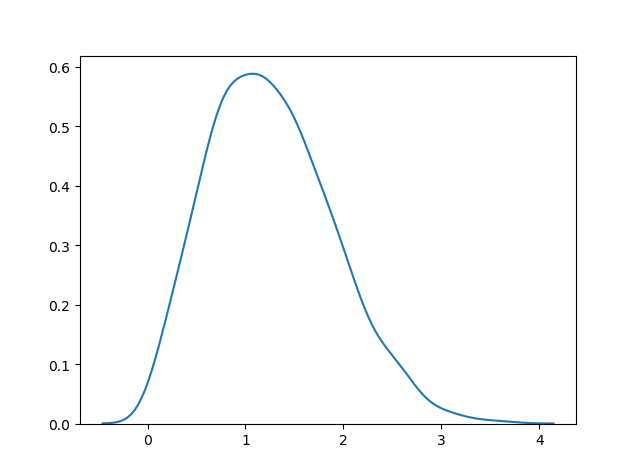THE WORLD'S LARGEST WEB DEVELOPER SITE

# Rayleigh Distribution

## Rayleigh Distribution

Rayleigh distribution is used in signal processing.

It has two parameters:

`scale` - (standard deviation) decides how flat the distribution will be default 1.0).

`size` - The shape of the returned array.

### Example

Draw out a sample for rayleigh distribution with scale of 2 with size 2x3:

from numpy import random

x = random.rayleigh(scale=2, size=(2, 3))

print(x)
Try it Yourself »

## Visualization of Rayleigh Distribution

### Example

from numpy import random
import matplotlib.pyplot as plt
import seaborn as sns

sns.distplot(random.rayleigh(size=1000), hist=False)

plt.show()

### ResultTry it Yourself »

## Similarity Between Rayleigh and Chi Square Distribution

At unit stddev the and 2 degrees of freedom rayleigh and chi square represent the same distributions.